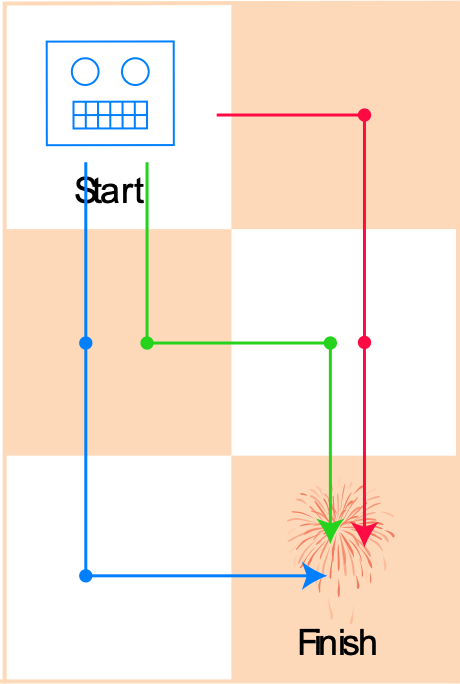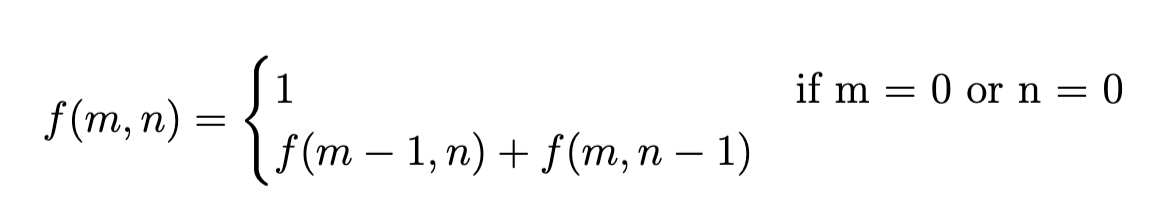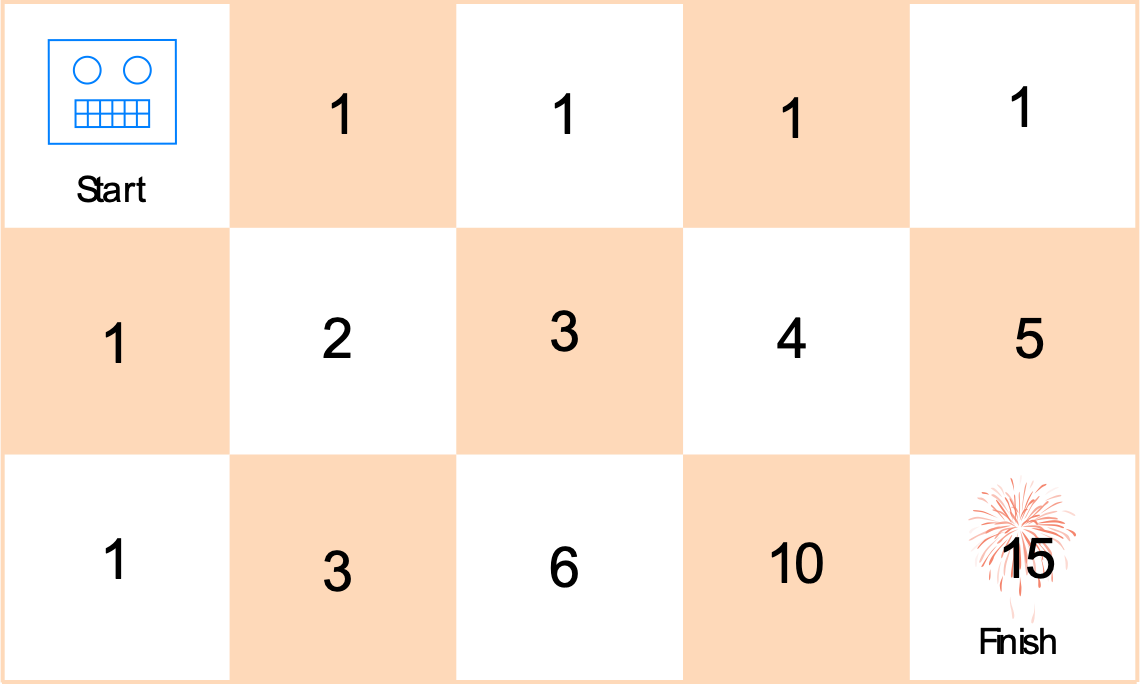# Unique Robot Paths

Today I’m going to go through a fun algorithm problem called Unique Robot Paths. Here’s the problem statement:

A robot is located at the top left corner of a m x n grid. Where m is the number of rows and n is the number of columns. The robot can only move either down or to the right. The robot’s destination is the bottom-right corner of the grid.

How many possible unique paths exist?One way to solve the problem is to recursively count all paths. However, the time complexity this solution is exponential, O(2^n).

## Can we do better?

Since this problem has these 2 important properties.

1. Overlapping sub-problems
2. Optimal substructure

These 2 properties allow us to use Dynamic Programming to solve this problem.

Let’s consider a smaller grid that is 3 x 2 in size.There are 3 ways to get to the bottom right. If we break the problem down, we can see how we can arrive at the answer.

Because the robot can only move down or right, the robot can only be one way to move to the right cell, and from there, 1 way to move to the bottom cell. This is one path to reach the destination.

Another path the robot could take, is the robot could move down 1 cell from the starting point and from there, move 1 space to the right.

And the third path the robot could take, is the to first move down 1 cell, move right 1 cell, and then finally, move down 1 cell to the finish.If you take a look at the grid, there’s a pattern — the number of ways to get to a certain cell equals the number of ways to get to its left cell PLUS the number of ways to get to the cell above it. Or expressed as an equation:So from his pattern, we can derive a solution.Going back to the initial problem with the 3 x 5 grid. Let’s fill out this grid and find the solution!

We know for the top row of cells there is only 1 way to reach each of the cells (m=0), and also the same is true for the left most column (n=0).From here, it’s easy to fill out the rest of the cells.So for a 5 x 3 grid, there are 15 unique paths that the robot can take.

### Let’s code up a solution in JavaScript!

``````/**
* @param {number} m
* @param {number} n
* @return {number}
*/
var uniquePaths = function(m, n) {
let storage = new Array(m).fill(new Array(n));

// fill the left most column of the storage matrix with 1's
for(let i=0; I < m; i++) {
storage[i] = 1;
}

// fill the top most row of the storage matrix with 1's
for(let j=0; j < n; j++) {
storage[j] = 1;
}

// iterate through the rest of the matrix and fill up the values
for(let i=1; I < m; i++) {
for(let j=1; j < n; j++) {
storage[i][j] = storage[i-1][j] + storage[i][j-1];
}
}

// return the bottom right cell's value
return storage[m-1][n-1];
};
``````

The run time for this solution is O(m x n) and the space complexity is also O(m x n) . A dramatic time complexity reduction for space trade-off. The above solution is intuitive, but there is a further optimization that reduces the space complexity to O(m).

``````/**
* @param {number} m
* @param {number} n
* @return {number}
*/
var uniquePaths = function(m, n) {
let storage = new Array(n).fill(0);

storage = 1;

for(let i=0; i < m; i++) {
for(let j=1; j < n; j++) {
storage[j] += storage[j-1];
}
}

return storage[n-1];
};
``````

Found this useful? Have a suggestion? Get in touch at `blog@hocnest.com`.